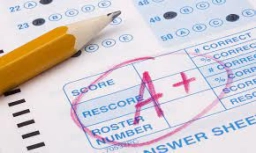# Testing students

Giving a test to a group of students, the grades and gender are summarized below.

A B C Total
Male 20 6 19 45
Female 17 18 13 48
Total 37 24 32 93

If one student is chosen at random,

a) Find the probability that the student got a B.

b) Find the probability that the student was female AND got a "C":

c) Find the probability that the student was female OR got a "B":

d) If one student is chosen at random, find the probability that the student got a 'B' GIVEN they are male

a =  0.2581
b =  0.1398
c =  0.7204
d =  0.1333

### Step-by-step explanation:Did you find an error or inaccuracy? Feel free to write us. Thank you!

Tips for related online calculators
Would you like to compute the count of combinations?